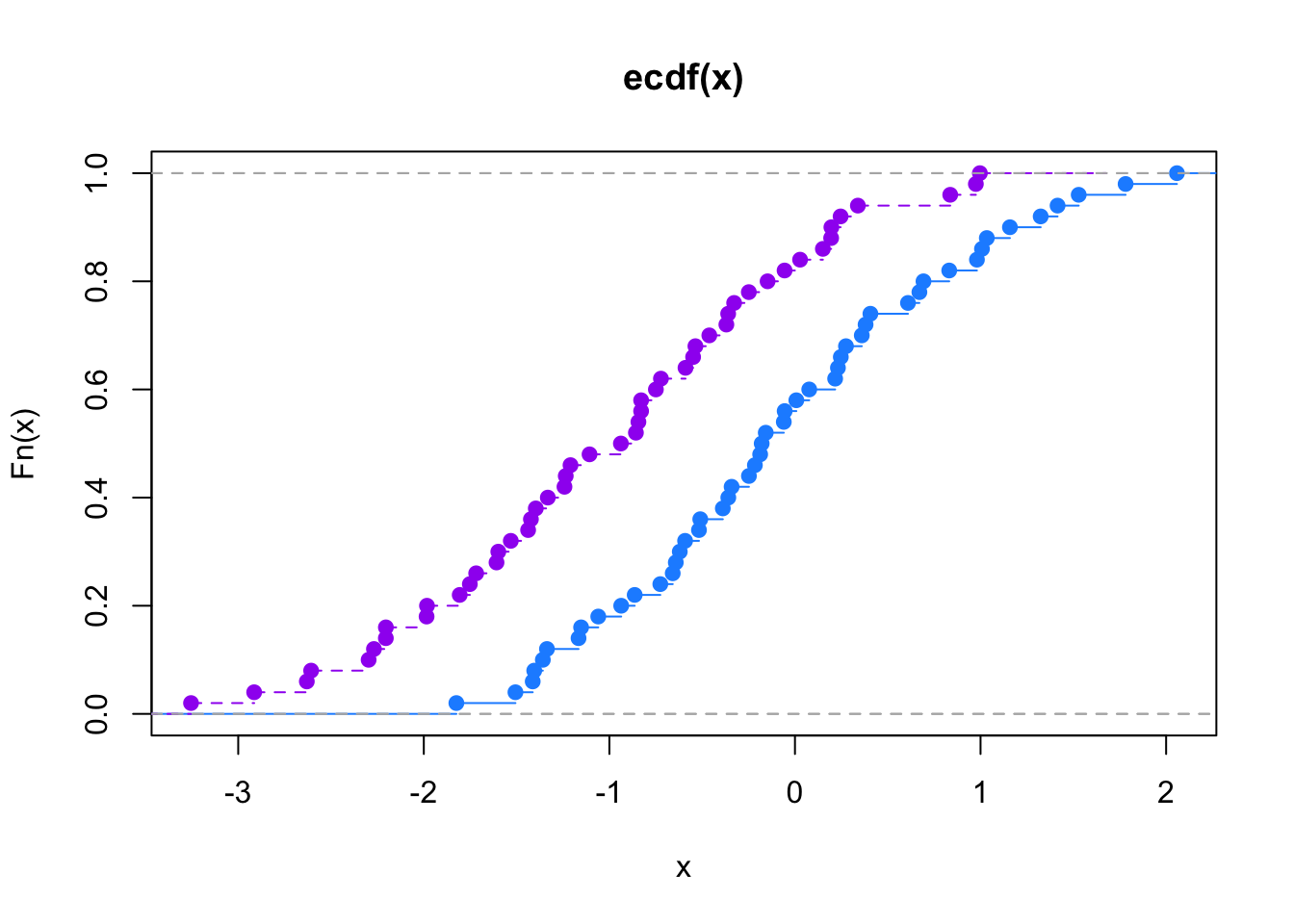### Usage and interpretation

In statistics, the Kolmogorov-Smirnov test (K-S test or KS test) is a nonparametric test of the equality of continuous, one-dimensional probability distributions that can be used to compare a sample with a reference probability distribution (one-sample K-S test), or to compare two samples (two-sample K-S test). The Kolmogorov-Smirnov statistic quantifies a distance between the empirical distribution function of the sample and the cumulative distribution function of the reference distribution, or between the empirical distribution functions of two samples.

The empirical distribution function $$F_n$$ for n iid observations $$X_i$$ is defined as $$F_n(x)={1 \over n}\sum_{i=1}^n I_{[-\infty,x]}(X_i)$$

where $$I_{[-\infty,x]}(X_i)$$ is the indicator function, equal to 1 if $$X_i \le x$$ and equal to 0 otherwise. $$D_n= \sup_x |F_n(x)-F(x)|$$

### Computational Programming Methods

In SAS, the Kolmogorov–Smirnov test is implemented in PROC NPAR1WAY. The discretized KS test is implemented in the ks.test() function in the dgof package of the R project for statistical computing. In Stata, the command ksmirnov performs a Kolmogorov–Smirnov test. Source: https://en.wikipedia.org/wiki/Kolmogorov%E2%80%93Smirnov_test

### Empirical Distribution Function

The empirical distribution function is the distribution function associated with the empirical measure of the sample. This cumulative distribution function is a step function that jumps up by 1/n at each of the n data points. The empirical distribution function estimates the cumulative distribution function underlying of the points in the sample and converges with probability 1 according to the Glivenko–Cantelli theorem. Source: https://en.wikipedia.org/wiki/Empirical_distribution_function

### “hello world” Example in R

From these simple examples we are able to determine if values come from the same distribution ($$H_o$$) or if they come from different distributions ($$H_a$$) and if the KS statisitic happend by random chance based on the p-value in either accepting the $$H_o$$ or failing to reject the $$H_o$$, and accept the $$H_a$$ (two-sample test). In a business context for the mix shift analysis shiny project if the two samples, being the pre-period and post-period are exhibiting a KS Statistic value that indicates they are from the same distribution then there has not been a statistically difference between those groups, which could be expected and/or prefered if the desire is to not cause radical shifts in expsoure between class plan iterations. If the business need expects there to be an appropriate amont of exposure difference then the KS statisitic should show that the two groups come from different distribution.

The lower the KS “D” statistic value to zero, equates to a more probable scenario that the two samples came from the same distribution (i.e. no change between pre-period and post-period). If the KS “D” statistic value is higher to 1, then that equates to a more probable scenario that the two sames did not come from the same distribution (i.e. no change between pre-period and post-period).

# this is a different implementation from the {stats} version
library(dgof)

x <- rnorm(50)
y <- runif(30)
# Do x and y come from the same distribution?
ks.test(x, y)
##
##  Two-sample Kolmogorov-Smirnov test
##
## data:  x and y
## D = 0.58, p-value = 2.381e-06
## alternative hypothesis: two-sided
# test if x is stochastically larger than x2
x2 <- rnorm(50, -1)
plot(ecdf(x), xlim=range(c(x, x2)), col="dodgerblue")
plot(ecdf(x2), add=TRUE, lty="dashed", col="purple")t.test(x, x2, alternative="g")
##
##  Welch Two Sample t-test
##
## data:  x and x2
## t = 4.8436, df = 97.299, p-value = 2.405e-06
## alternative hypothesis: true difference in means is greater than 0
## 95 percent confidence interval:
##  0.6178177       Inf
## sample estimates:
##   mean of x   mean of y
## -0.06381104 -1.00397293
wilcox.test(x, x2, alternative="g")
##
##  Wilcoxon rank sum test with continuity correction
##
## data:  x and x2
## W = 1870, p-value = 9.742e-06
## alternative hypothesis: true location shift is greater than 0
ks.test(x, x2, alternative="l")
##
##  Two-sample Kolmogorov-Smirnov test
##
## data:  x and x2
## D^- = 0.38, p-value = 0.0007318
## alternative hypothesis: the CDF of x lies below that of y